# Author an algorithmic question

Algorithmic questions are static question templates that with variables inserted into them to make them algorithmic.

NOTE: Algorithmic content is authored using the Möbius language with a specific accepted syntax. Check out the Follow Algorithm Editor syntax rules help topic for more help on the accepted syntax during algorithm authoring that's unique to Möbius. You can also view the Use proper math syntax during question authoring help topic for additional details on how to define your correct answer with proper syntax. In addition, whenever Maple calls are used within algorithms, Maple syntax is required.

NOTE: Variable names must start with the \$ (dollar sign) character (Example\$m). To use the actual dollar sign character in your question statement or response area, use \\$. The use of the backslash character (\) is reserved as an escape character in algorithmic command syntax. Use \\ to insert the actual backslash character. Check out Follow Algorithm Editor syntax rules.

These variables are algorithmic and are assigned random values (according to defined ranges and conditions) each time the question template is used in an activity.

Therefore, algorithmic questions generate multiple question permutations from a single question template each time the question is reused.

The numbers and wording (depending on how the algorithmic variable is defined) present new, randomly-determined values each time an activity is attempted.

Students will see different variable values each time a new attempt is launched (even if a student is launching a subsequent attempt at the same activity).

TIP: Enable the Keep Variation property within the Assignment Editor when creating your homework or quiz assignment so that the student can return and rework an assignment with the same algorithmic variable values for each repeated attempt. Check out step 3 of Define assignment properties.

As well, when multiple students launch the same activity, each student will be shown different values for the same algorithmic question of the activity.

The variable data that's created for a particular student's attempt persists throughout the activity session—even when the student navigates between questions—until the student's attempt is submitted for grading.

NOTE: Algorithmic variable values are recorded permanently in the Gradebook. When students or instructors review the graded activity, the exact variable values that were included in the attempt being investigated are maintained.

Algorithmic variables can be added to questions using the Question Editor in 2 ways:

NOTE: Both methods use the same syntax for commands, but with the Algorithm Designer, the syntax is entered for you once you've defined the variables and values.

NOTE: Algorithmic variables can be used anywhere within any of the question types (including adaptive questions). However, algorithmic variables can't be used to define the correct answer for sketching questions and free body diagram questions.

Once an algorithmic variable is defined, it can also be referenced by other parts of the algorithmic question:

• Hints
• Content feedback

NOTE: Other content types (Example — text, Math Apps, Interactive Narratives) in both lessons and assignments can also use algorithmic variables.

## To author an algorithmic question

Algorithmic questions are authored the same way any non-algorithmic question is authored from the Question Editor.

The static question (a question where the variables have fixed, defined values) is generally authored first, and then the algorithmic variable(s) can be inserted after.

To author an algorithmic question from the Question Editor:

TIP: You can also perform the following tasks using this same procedure:

• Transform an existing static question into an algorithmic question.
• Make edits to an existing algorithmic question.
1. Author a static question using the Question Editor as you normally would. Check out Author a question for reminders of this process.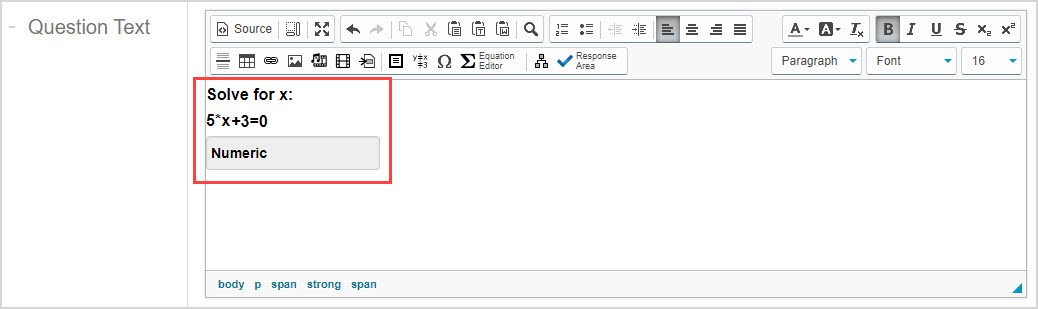1. Click expand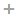to expand the Algorithm pane of the Question Editor.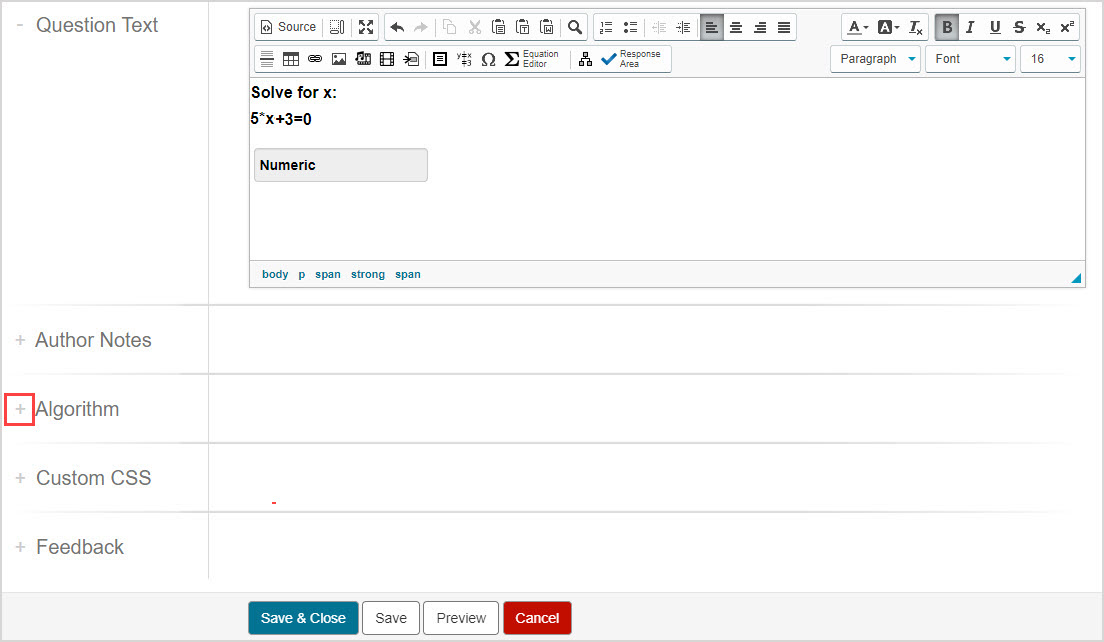NOTE: When working with a question in the Lesson Editor or Assignment Editor, the Algorithm Editor is stored within a panel of the Question Editor. Click the Algorithm tab to reveal the Algorithm Editor.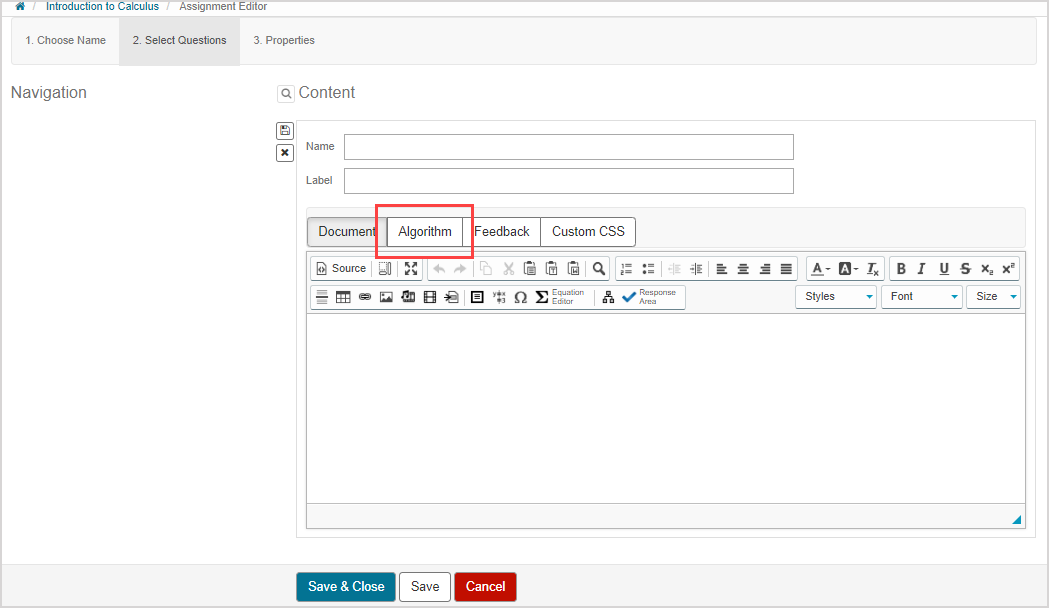1. The Algorithm Editor is revealed.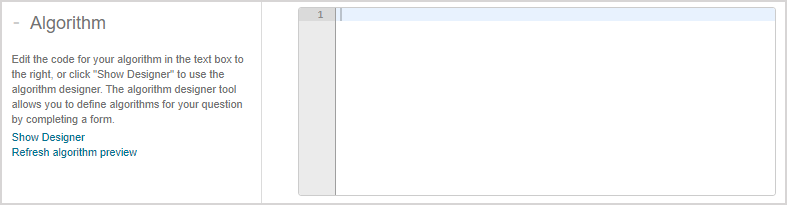1. You can now define algorithmic command lines.

NOTE: Algorithmic commands within the Algorithm Editor function the same, regardless of whether the commands are manually entered or structured for you when you use the Algorithm Designer.

TIP: Be sure to click save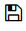after you've completed your algorithmic commands if you are working with an algorithm within the Lesson or Assignment Editors.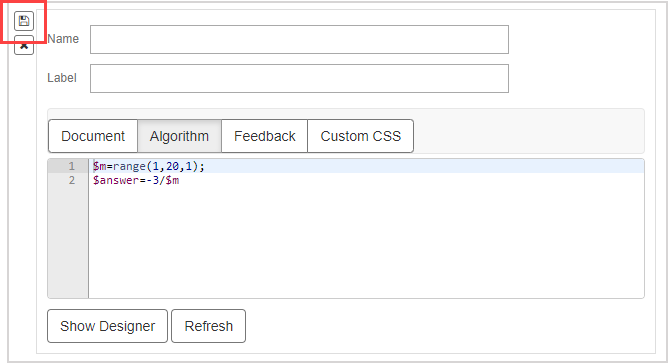1. After creating your algorithm in step 3, navigate back to the Question Text pane and replace the fixed values in the question with the variable names that you defined in your algorithm. Example — The 5 in the static question of 5*x+3=0 is replaced with \$m which is defined by the function \$m=range(1,20,1);.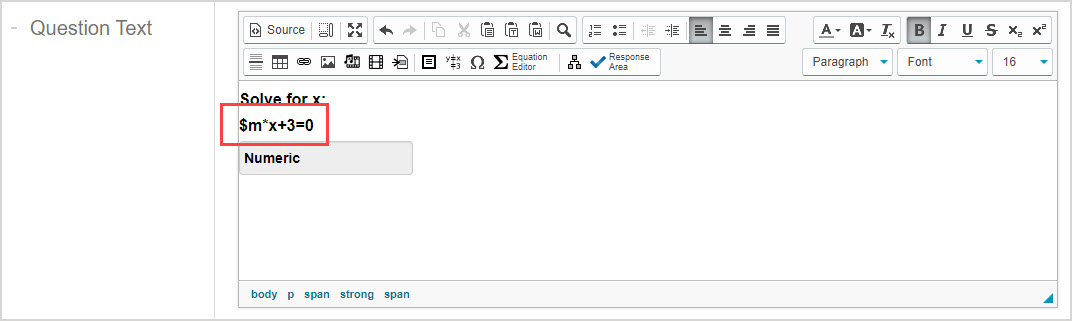IMPORTANT: Variable names must start with the \$ (dollar sign) character. Example\$m

TIP: Use \\$ to insert the actual dollar sign character in your question statement or response area. The use of the backslash character (\) is reserved as an escape character in algorithmic command syntax. Use \\ to insert the actual backslash character. Check out Follow Algorithm Editor syntax rules.

NOTE: When working with a question in the Lesson or Assignment Editors, the Question Text pane is stored within a panel of the Question Editor. Click the Document tab to reveal the question text.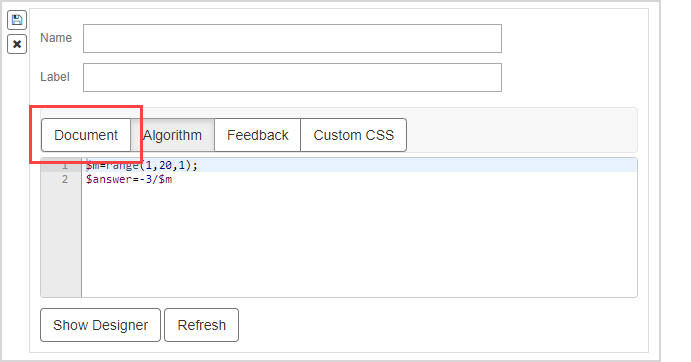1.  Click Save to save your changes to the question.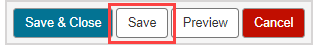TIP: Click Preview to view your question that now includes an algorithmic variable. Each time the question is previewed, the algorithmic variable is replaced with a new value. Example — Viewing the question preview 3 different times will display 3 different values for your algorithmic variable.If you're previewing your algorithmic question from the Lesson Editor, click Try Another to see the algorithmic variable replaced with new values each time the question is viewed.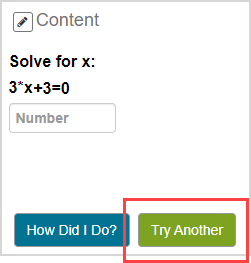1. Your question is now an algorithmic question and can be incorporated into your activities. Algorithmic questions are added to activities the same way any other type of content is imported or added. Check out Import lesson content or Import assignment questions.

## Use the algorithmic variable elsewhere

After the algorithmic variable is defined, you can apply it to other parts of a question:

• Hints
• Content feedback

NOTE: Other content types (Example — text, Math Apps, Interactive Narratives) in both lessons and assignments can also use algorithmic variables in a similar manner to how they're applied to questions. Use the content-specific Editor to insert algorithmic variables to these other content types.

While authoring a question and defining the correct response, you can include the algorithmic variable in the answer field within an expression to give the correct answer:TIP: A correct answer variable can be used to calculate the correct answer and is defined by an expression including the algorithmic variable of your question. This correct answer variable is entered into the Question Editor's Edit Response Area window instead of the expression that's used to calculate the correct answer. This is ideal to ensure that your correct answer behaves as expected. As well, if the correct answer calculation is complex, it's easier to define it in the larger Algorithm Editor entry field. To use a correct answer variable in the Algorithm Editor:

1. Define a variable for the correct answer.1. Define the expression that will give the correct answer based on your question and the algorithmic variable that you've used in the question.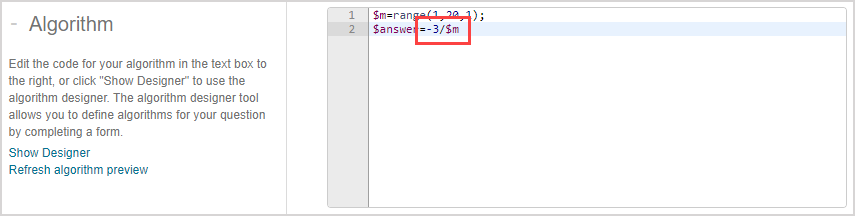1. Click Refresh algorithm preview (or Refresh if using the Algorithm Designer in the Lesson or Assignment Editors) as many times as needed to ensure that the values that are derived for your defined correct answer variable display as expected.1. The correct answer will be displayed for your correct answer variable based on the algorithmic variables defined in your algorithm and the expression defined for calculating the correct answer. Ensure that the values that are calculated for your correct answer variable display as expected.1. Double-click the response area field of your question text to edit the question's response area.1. Enter your correct answer variable in the answer field of the Question Editor's Edit Response Area window so that the question references this expression to calculate the correct answer and compares it to the student's submitted answer.1. Click Update for your question to use the defined correct answer variable.### Hints

While authoring a hint, simply include the algorithmic variable in the hint text: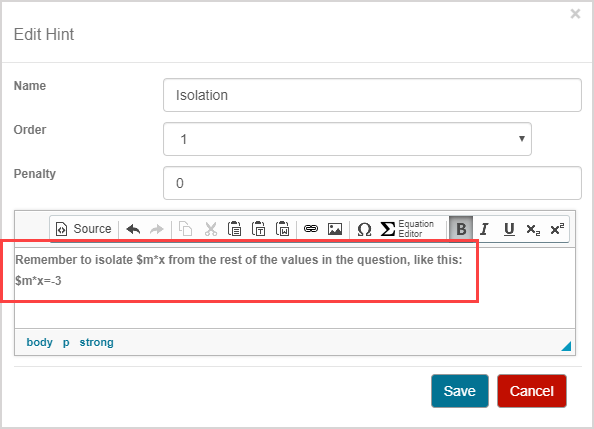When the hint is viewed, the algorithmic variable will update to represent the value being viewed in the question: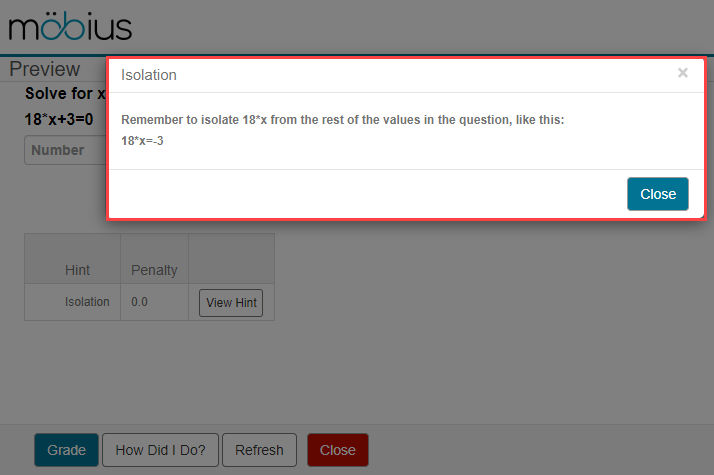### Content feedback

While authoring content feedback, simply include the algorithmic variable in the content feedback text: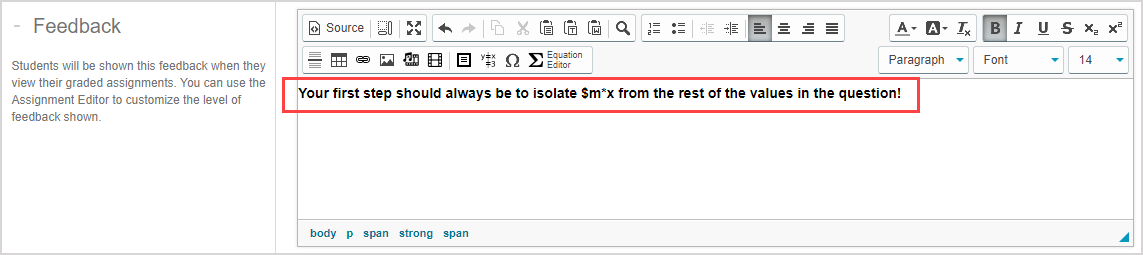When the content feedback is viewed, the algorithmic variable will update to represent the value being viewed in the question: